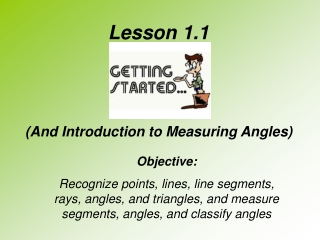DownloadDownload PresentationLesson 1.1 (And Introduction to Measuring Angles)

# Lesson 1.1 (And Introduction to Measuring Angles)

Télécharger la présentation## Lesson 1.1 (And Introduction to Measuring Angles)

- - - - - - - - - - - - - - - - - - - - - - - - - - - E N D - - - - - - - - - - - - - - - - - - - - - - - - - - -
##### Presentation Transcript

1. Lesson 1.1 (And Introduction to Measuring Angles) Objective: Recognize points, lines, line segments, rays, angles, and triangles, and measure segments, angles, and classify angles

2. Basic Definitions Points: Represented by capital letters (Draw 3 points and label them) Lines: Lines are made up of points and are straight. Arrows are drawn on the ends to show that the lines extend infinitely far in both directions.

3. Basic Definitions More on Lines: Lines can be named based on any two points. Let’s take a look at an example: Name the line in 3 different ways. B l A

4. Basic Definitions Number Line: A number line is formed when a numerical value is assigned to each point on a line. Example: Draw a number line from -2 to 3 using one tick mark per integer.

5. Basic Definitions Line Segment: Like lines, segments are made up of points and are straight, however, segments have a definite beginning and end. Line Segments are named by their endpoints. Examples: Name the following line segments P S R X Q

6. Basic Definitions Rays: Like lines and segments, rays are made up of points and are straight. A ray differs from a line or segment in that it begins at an endpoint and extends infinitely far in only one direction. Examples: M D J K P C L

7. Note: It is important to keep in mind that when we name a ray, we name the endpoint first! This makes it clear as to where the ray begins. Name the following rays: M D J P K C L

8. Basic Definitions Angles: Two rays that have the same endpoint form an angle. Def. An Angle is made up of two rays with a common endpoint. This point is called the vertex of the angle. The rays are called sides of the angle. Examples: P T B 1 A 2 2 C H S

9. Naming Angles When naming an angle with 3 letters you must name the vertex in the middle! Every time…no exceptions! Examples: Name the following angles P T B 1 A 2 2 C H S

10. Triangles A A triangle has three segments as its sides and three angles at each of its vertices. How could you name this triangle? B C

11. Unions and Intersections Unions and Intersections are a way to help us see where certain pieces of an object overlap, and what they form when pieced together. In terms of a triangle: The triangle is the union (U) of three segments: The intersection (∩) of any two sides is a vertex of the triangle: A B C

12. Example #1 a. b. c. A E B C m D

13. Example #2 Draw a diagram in which the intersection of with is segment

14. Measuring Segments Segments can be measured using tools such as rulers or meter sticks, and often, segments found on number lines can be measured by subtracting the ending and starting value. Example: Q P

15. Measuring Angles Angles are measured using Protractors, and in this course we will be measuring angles in degrees. The measure (or size) of an angle is the amount of turning you would do if you were at the vertex, looking along one side, and then turned to look along the other side. (A surveyor’s transit works in a similar way!)

16. Classifying Angles by Size Angles can be classified into four groups:

17. Homework Lesson 1.1 Worksheet